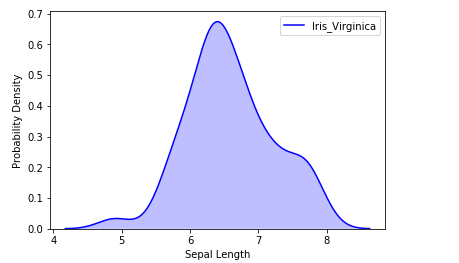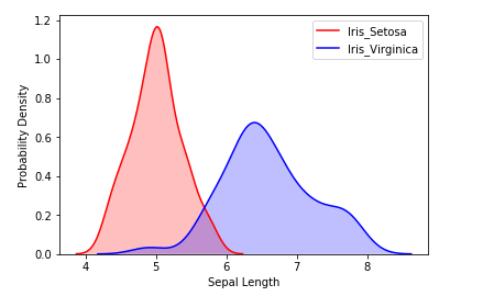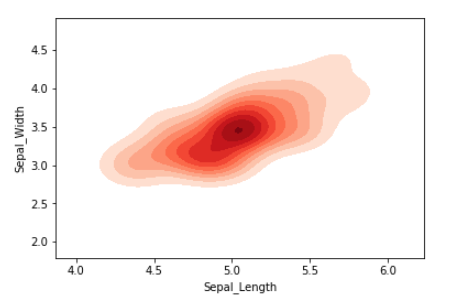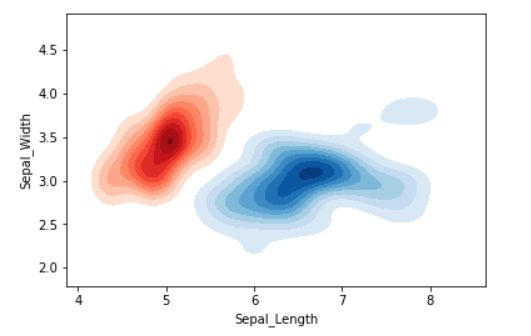# KDE Plot Visualization with Pandas and Seaborn

KDE Plot described as Kernel Density Estimate is used for visualizing the Probability Density of a continuous variable. It depicts the probability density at different values in a continuous variable. We can also plot a single graph for multiple samples which helps in more efficient data visualization.

In this article, we will be using Iris Dataset and KDE Plot to visualize the insights of the dataset.

1. Attributes : Petal_Length (cm), Petal_Width (cm), Sepal_Length (cm), Sepal_Width(cm)
2. Target : Iris_Virginica, Iris_Setosa, Iris_Vercicolor
3. Number of Instances : 150

One-Dimensional KDE Plot :

We can visualize the probability distribution of a sample against a single continuous attribute.

 `# importing the required libraries ` `from` `sklearn ``import` `datasets ` `import` `pandas as pd ` `import` `seaborn as sns ` `import` `matplotlib.pyplot as plt ` `%``matplotlib inline ` ` `  `# Setting up the Data Frame ` `iris ``=` `datasets.load_iris() ` ` `  `iris_df ``=` `pd.DataFrame(iris.data, columns``=``[``'Sepal_Length'``, ` `                      ``'Sepal_Width'``, ``'Patal_Length'``, ``'Petal_Width'``]) ` ` `  `iris_df[``'Target'``] ``=` `iris.target ` ` `  `iris_df[``'Target'``].replace([``0``], ``'Iris_Setosa'``, inplace``=``True``) ` `iris_df[``'Target'``].replace([``1``], ``'Iris_Vercicolor'``, inplace``=``True``) ` `iris_df[``'Target'``].replace([``2``], ``'Iris_Virginica'``, inplace``=``True``) ` ` `  `# Plotting the KDE Plot ` `sns.kdeplot(iris_df.loc[(iris_df[``'Target'``]``=``=``'Iris_Virginica'``), ` `            ``'Sepal_Length'``], color``=``'b'``, shade``=``True``, Label``=``'Iris_Virginica'``) ` ` `  `# Setting the X and Y Label ` `plt.xlabel(``'Sepal Length'``) ` `plt.ylabel(``'Probability Density'``) `

Output:We can also visualize the probability distribution of multiple samples in a single plot.

 `# Plotting the KDE Plot ` `sns.kdeplot(iris_df.loc[(iris_df[``'Target'``]``=``=``'Iris_Setosa'``), ` `            ``'Sepal_Length'``], color``=``'r'``, shade``=``True``, Label``=``'Iris_Setosa'``) ` ` `  `sns.kdeplot(iris_df.loc[(iris_df[``'Target'``]``=``=``'Iris_Virginica'``),  ` `            ``'Sepal_Length'``], color``=``'b'``, shade``=``True``, Label``=``'Iris_Virginica'``) ` ` `  `plt.xlabel(``'Sepal Length'``) ` `plt.ylabel(``'Probability Density'``) `

Output:Two-Dimensional KDE Plot :

We can visualize the probability distribution of a sample against multiple continuous attributes.

 `# Setting up the samples ` `iris_setosa ``=` `iris_df.query(``"Target=='Iris_Setosa'"``) ` `iris_virginica ``=` `iris_df.query(``"Target=='Iris_Virginica'"``) ` ` `  `# Plotting the KDE Plot ` `sns.kdeplot(iris_setosa[``'Sepal_Length'``],  ` `            ``iris_setosa[``'Sepal_Width'``], ` `            ``color``=``'r'``, shade``=``True``, Label``=``'Iris_Setosa'``, ` `            ``cmap``=``"Reds"``, shade_lowest``=``False``) `

Output:We can also visualize the probability distribution of multiple samples in a single plot.

 `# Plotting the KDE Plot ` `sns.kdeplot(iris_setosa[``'Sepal_Length'``], ` `            ``iris_setosa[``'Sepal_Width'``], ` `            ``color``=``'r'``, shade``=``True``, Label``=``'Iris_Setosa'``, ` `            ``cmap``=``"Reds"``, shade_lowest``=``False``) ` ` `  `sns.kdeplot(iris_virginica[``'Sepal_Length'``],  ` `            ``iris_virginica[``'Sepal_Width'``], color``=``'b'``, ` `            ``shade``=``True``, Label``=``'Iris_Virginica'``, ` `            ``cmap``=``"Blues"``, shade_lowest``=``False``) `

Output:Attention geek! Strengthen your foundations with the Python Programming Foundation Course and learn the basics.

To begin with, your interview preparations Enhance your Data Structures concepts with the Python DS Course.

My Personal Notes arrow_drop_upCompetitive Programmer

If you like GeeksforGeeks and would like to contribute, you can also write an article using contribute.geeksforgeeks.org or mail your article to contribute@geeksforgeeks.org. See your article appearing on the GeeksforGeeks main page and help other Geeks.

Please Improve this article if you find anything incorrect by clicking on the "Improve Article" button below.

Article Tags :
Practice Tags :

Be the First to upvote.

Please write to us at contribute@geeksforgeeks.org to report any issue with the above content.